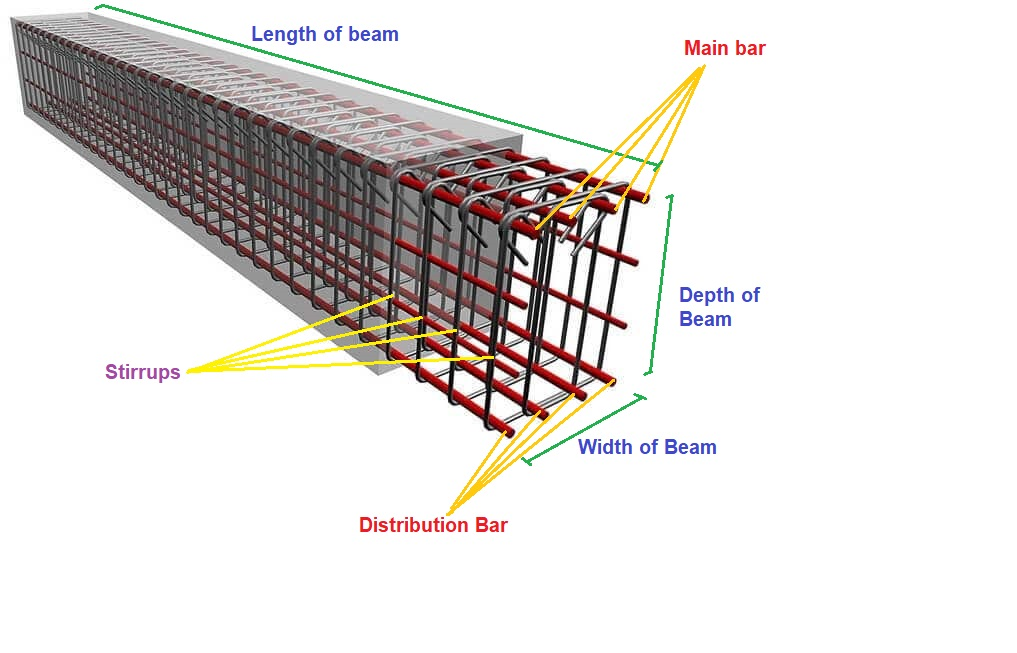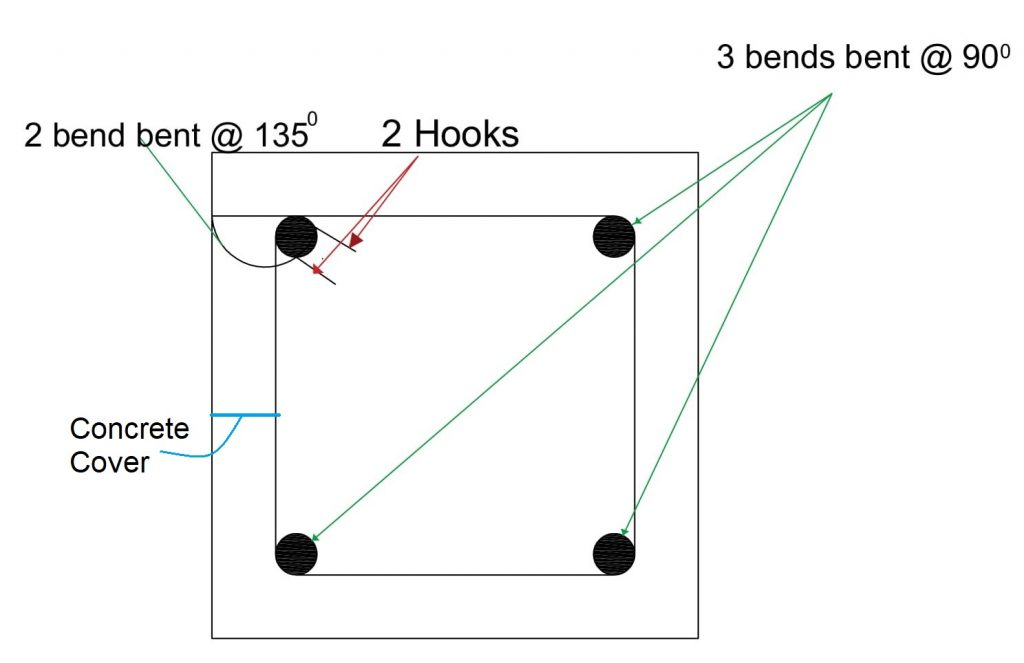Work Hours
Monday to Friday: 7AM - 7PM
Weekend: 10AM - 5PM

# Steel Weight Calculator For Beam

## Calculations for Steel Calculator for BeamAssumptions for Example

• There are 2 types of bars in a Beam: Main Bar & Distribution Bar
• Main(Top) Bar Diameter = 16 mm
• Number of Main(Top) Bars = 3
• Distribution(Bottom) Bar Diameter = 20 mm
• Number of Distribution(Bottom) Bars = 3
• Length of Beam = 4500 mm
• Width of Beam = 230 mm
• Depth of Beam = 600 mm
• Diameter of Stirrup(Ring) = 8 mm
• C/C distance of ring(mm) at supports= 100 mm
• C/C distance of ring(mm) at mid supports= 150 mm
• Concrete Cover = 25 mm
• We are using Steel grade of fe500

Calculation Procedure

• Step 1: Calculation of cutting length for Main(Top) & Distribution(Bottom) Bar’s
Cutting Length  of Main Bar = Length + Development Length(on 2 sides) – Bend(on 2 ends) – clear cover
Cutting Length  of Distribution Bar = Length + Development Length(on 2 sides) – Bend(on 2 ends) – clear cover
Here , Development Length = 50D & Bend =2D ; where D= Diameter of Bar
Cutting Length of Main Bar = 4500 + 2x50x16 – 2x2x16 – 2×25
= 5986 mm
Cutting Length of Distribution Bar = 4500 + 2x50x20 – 2x2x20 – 2×25
= 6370 mm
• Step 2: Calculate number of stirrup
Number of Stirrups required = 2xStirrup(support zone) + Stirrup(mid support zone)
= 2[(L/3)/spacing at support + 1] + [(L/3)/spacing at mid support + 1]
= 2[(4500/3)/100 + 1] + [(4500/3)/150 + 1]
= 43
• Step 3: Calculate cutting Length of Stirrup
Cutting length of Stirrup = Perimeter of stirrup + Number of Bends + Number of Hooks
= 2(a+b) + 3 numbers of 90 degree bends + 2 numbers of hooks
= 2(a+b) + 3x2xd + 2x2xd
Here, a & b are Width & Depth of beam ; d is the diameter of stirrup
Cutting length of stirrup = 2(230 + 600) + 3x2x8 + 2x2x8
= 1740 mm
• Step 4: Total Length Calculation
Total Length of Main(top) Bar = 3 x 5986
= 17958 mm = 18 m
Total Length of Distribution(bottom) Bar = 3 x 6370
= 19110 mm = 20 m
Total Length of Stirrup Bar = 43 x 1740
= 74820 mm = 75 m
• Step 5:  Calculation of Steel Weight
Weight of the bar as per their length and diameter by following Formula: –
Unit weight formula for kg per metre =(D2/163) × L kg/m
Unit weight for feet per metre = (D2/ 533) × L kg/ft
L is the required length of Steel bar of Diameter D
Weight of Main Bar = (162/163) x 18 = 28.27 Kg
Weight of Distribution Bar = (202/163) x 20 = 40.08 Kg
Weight of Stirrup Bar = (82/163) x 75 = 29.45 Kg

Final Result

Total Steel Weight =  28.27 + 40.08 + 29.45 = 97.8kg

Try Onsite, #1 Tool For Site Tracking